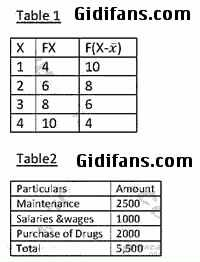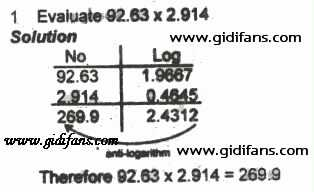SYMBOL CLASS FOR MATHS,PHYSICS, CHEMISTRY, ACCOUNT,

SCROLL DOWN TO VIEW MORE

(1) / means division or divide
Examples:
2/2 means 2/2
2 whole no 3/4 means 2¾
M=wp+(1/3)yp^2 means m=wp + ¾y2

(2) Log means logarithm
Example: (8) Log5 (base 8) means Log85

(3) * means multiplication
Example: 2*2 means 2x2

(4) ^ means Raise to power
Examples:
3^(-1) means 3-1
5^(2) means 52
30cm^(-2) means 30cm-2

(5) Tita means θ
Example: Tan tita means tan θ

(6) Pie means π
Example: Pie R sqr h means πr2h

(7) Base means subscript
Example: 2 Base 3 means A3

(8) Sqr root means √
Examples:
√3-√6+2√3 means Root 3-root6+2root 3
√(¾) means sqr root(3/4)

(9) bar means a dash on top a number of letter
Example: X bar means x̅

(10) ---(1) means equation 1

(11) Proportional means ∝
Example: R is proportional to 3/4rut m means R ∝ ¾

(12) Alpha means α

(13) Beta means β

(14) Gamma means γ

(15) cube root means ∛

(16) Mu means μ

(17) Rho means ρ

(18) Delta means δ

(19) Sigma means σ

(20) Tau means τ

(21) Ohm means Ω

(22) Lamda means λ

(23) Omega means ω

(24) Intersection means ∩
Example: B intersection C means B∩C

(25) Union means U
Example: B union C means BUC

(26) Factorial means !

(27) complements in sets means ‘

(28) (aq),(g),(l),(s) is used in chemistry to show the state of matter in equations

(29) Equivalent to means ≡

(30) Not equal to means ≠

(31) Quotation means “ ”

(32) Less or equal to means ≤

(33) Greater or equal to ≥
Example: (3/4)>=(1/2) means ¾ ≥ ½

(34) Plus or minus (or +-) means ±
Example: (3/4)<=(1/2) means ¾ ≤ ½

(35) Element of means ∈
Example: 2 belongs to X or 2 is a member of X means 2∈X

(36) Percent or percentage means %

(37) Degrees Fahrenheit means ℉

(38) Degrees Celsius means ℃

(39) Universal set means ξ or U

(40) Null or empty set means ∅ or {}

(41) <RST means RŜT

(42) 2.S.F means 2 significant figures

(43) P=(2,3) means P={2,3} in sets

(44) Propotional to means ∝

(45) Therefore means ∴

(46) KNO(3)(s) means KNO3(s)

(47) NaCl=> Na^(+) + Cl^(-) means NaCl → Na++ Cl-

(48) Summation means Ʃ

(49) Parallel lines means ǁ

(50) Perpendicular lines means ˩

(51) Reversible equation means ↔

(52) Infinity means ∞

(53) Approximately means ~

(57) Approximately equal to ≅

Other Symbols
(1) File means ɸ
(2) Δ means Change or Triangle
(3) slope or differentiation means dy/dx or ∂y/∂x or δy/δx or Δy/Δx
(4) Parenthesis means …
(5) Naira means ₦
(6) Integral means ∫
(7) Almost equal to means ≈
(8) For all means ∀
(9) There exist means ∃
(10) Epsilon means ε
(11) Vertical dots means ⁞Table1 A table of this would be computer as follows X:1,2,3,4 FX:4,6,8,10 F(X-Xbar):10,8,6,4 Table2 A table like this would be computed as follows Particulars: maintenance,salaries &wages, Purchase of Drugs, total Amount: 2500,1000,2000,5500 Logarithm TableOrTabulate No:92.63,  Log:1.9667 No: 2.914,  Log: 0.4645 2.4312 Antilog = 269.9 Therefore 92.63*2.914=2.4312 Radiativity Diagrammeans (238)U(92)=>(234)Th(90) + (4)He(2)This is computed  as follows (1)(-b± sqr rut(b^2-4ac))/2a (2)Sqr root ((2Qh)/100) (3)Xbar=Efx/ef (4)Summation (n=1 to infinity )P(n) (5)S.D= Sqr Rut ((Ef|x-xbar|^2))/Ef (6)R is proportional to1/ sqr rut